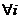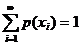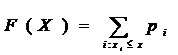# Statistics Assignment Help With Random Variable And Discrete Random Variable

If a random variable takes at most a countable number of values, it is called a discrete random variable. In other words, a real valued function defined on a discrete sample space is called a discrete random variable.### 3.3.1 Probability mass function:

Suppose X is a one – dimensional discrete random variable taking at most a countably infinite number of values x1, x2……… with each possible outcome xi, we associate a number pi = P(X =xi) = p(xi), called the probability of xi. the number p(xi); I = 1, 2,…….. must satisfy the following conditions:

1. (i) P(xi) ≥02. (ii)This function p is called the probability mass function of the random variable X and the set { xi, p(xi)} is called the probability distribution (p.d.) of the r.v. X.

### 3.3.2 Discrete distribution function:

Suppose there are a countable number of points x1, x2,……… and numbers p≥0,. Such thatFor example if xi is just the integer i. F(x) is a “step function” having jump pi at I, and being constant between each pair of integers.